# 正弦定理

a sin A = b sin B = c sin C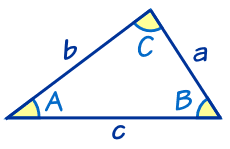a、b 和 c 是边。 A、B 和 C 是角。 （边 a 对着 角 A、 边 b 对着 角 B， 边 c 对着 角 C）。

## ？？？？？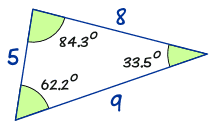a sin A = 8 sin(62.2°) = 8 0.885…… = 9.04…… b sin B = 5 sin(33.5°) = 5 0.552…… = 9.06…… c sin C = 9 sin(84.3°) = 9 0.995…… = 9.05……

（若我们的计算有完全的准确度，答案便会是完全相同的）。

a sin A = b sin B = c sin C

## 有什么用？

### 例子：求 边 "c" 的 长度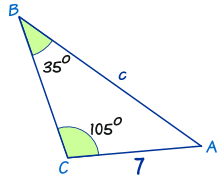正弦定理 a/sin A = b/sin B = c/sin C 代入已知值： a/sin A = 7/sin(35°) = c/sin(105°) 拿走 a/sin A （在这里没用）： 7/sin(35°) = c/sin(105°) 用代数来重排及解： 换边： c/sin(105°) = 7/sin(35°) 每边乘以 sin(105°)： c = ( 7 / sin(35°) ) × sin(105°) 计算： c = ( 7 / 0.574…… ) × 0.966…… 计算： c = 11.8 （保留一个小数位）

## 求未知角度

……但我们也可以用正弦定理来求未知角度

sin A a = sin B b = sin C c

### 例子：求 角 B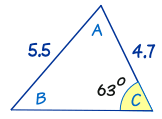开始： sin A / a = sin B / b = sin C / c 代入已知值： sin A / a = sin B / 4.7 = sin(63°) / 5.5 拿走 "sin A / a"： sin B / 4.7 = sin(63°) / 5.5 每边乘以 4.7： sin B = (sin(63°)/5.5) × 4.7 计算： sin B = 0.7614…… 反正弦： B = sin-1(0.7614……) B = 49.6°

## 有时会有两个答案！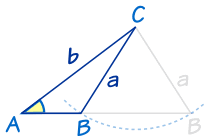假设我们知道 角 A 和 边 a 与 b。 我们可以把边 a 放在左边或右边（如图），从而得到两个结果（一个小三角形和一个大三角形） 两个答案都是对的！

### 例子：求 角 R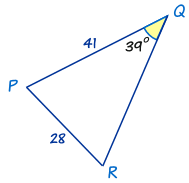开始： sin R / r = sin Q / q 代入已知值： sin R / 41 = sin(39°)/28 每边乘以 41： sin R = (sin(39°)/28) × 41 计算： sin R = 0.9215…… 反正弦： R = sin-1(0.9215……) R = 67.1°

180° - 67.1° = 112.9°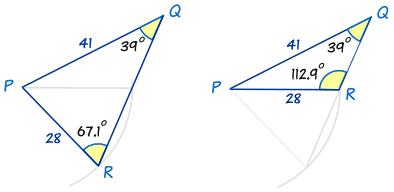• ……有时候会有两个答案（如上）
• ……有时候只有一个答案（如下）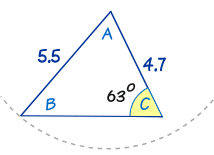我们以前也看过这个三角形。 你可以尝试把 "5.5" 长的边放到右边，但这样不会形成一个符合给定条件的三角形，所以也不会导致一个合理的答案。 故此，这算题只有一个答案。